## Introduction

Friction between sliding surfaces in living systems is often at levels that engineers can only envy. Thus, friction coefficients of order 10−3, at pressures exceeding 100 atm, typical of articulating cartilage surfaces in healthy human joints1, cannot be reproduced by any synthetic surfaces, and its molecular-level understanding remains elusive2. Over the past decade, the concept of hydration lubrication has been invoked to account for the extremely low sliding friction observed at high pressures between charged surfaces in high-salt solution3,4,5, or when coated by surfactants6,7,8, liposomes9 or hydrated polymer brushes10,11,12—boundary layers resembling those at articular cartilage surfaces12,13. According to this, hydration shells formed by water molecules are tenaciously attached to the charges they surround, and so cannot be easily squeezed out on compression14,15,16,17,18, yet are labile and so respond to shear in a fluid manner. However, there is little microscopic understanding of this mechanism, especially of the frictional dissipation within the subnanometre hydration shells that form its basic elements. This is because in all relevant studies to date, including refs 4, 5, 6, 7, 8, 9, 10, 11, the measured friction may have been dominated by other dissipation pathways, such as polymer chains disentangling past each other10,11 or distortion of sheared liposomes9.

The present study overcomes these limitations by trapping hydrated ions in the gap between molecularly smooth surfaces, and measuring the friction between them as they slide at pressures and shear rates one to two orders of magnitude higher than previously achieved3. This allows a clear separation of different dissipation regimes, and enables to isolate the dissipation arising from shear of the subnanometre hydration shells themselves, which are intrinsic to all hydration lubrication processes, from any system-dependent dissipation. Our results reveal that viscous dissipation within hydration shells—for the ions examined—is some 250-fold larger than the viscosity either of bulk water or of similarly confined non-hydration water. This sheds strong light on the origins of hydration lubrication, with implications both for aqueous boundary lubricants and for lubrication in biological systems.

## Results

### Normal surface forces

We use a surface force balance (SFB) with state-of-the-art resolution and sensitivity in measuring interactions, particularly shear forces, between curved, atomically smooth mica surfaces (mean radius of curvature R≈1 cm), across nanometre and subnanometre liquid films, as described in detail earlier3 (Methods) and schematically shown in the inset of Fig. 1. Here we measure the normal and shear forces, Fn(D) and Fs(D), respectively, between such surfaces at separation D, across highly pure aqueous solutions of ca 0.1 M NaNO3 or NaCl at mean pressures P and sliding velocities νs that are up to two orders of magnitude higher than in earlier studies3,19 (Methods). Figure 1 shows the normalized force profiles Fn(D)/R versus D across water (always taken as a control for system cleanliness before adding salt) and across the 0.1 M salt solutions, revealing the longer-ranged repulsion arising from counterion osmotic pressure18,20 down to D≈2 nm. At D<ca 2 nm, we observe the characteristic repulsion arising from the tightly bound hydration shells14,15,16,17,18 surrounding the Na+ counterions trapped between the negatively charged mica surfaces.Figure 1: Normal forces across confined hydrated ions.

### Shear forces across hydrated ions

To elucidate the nature of the frictional dissipation, we measured the dependence of the shear forces Fs on νs at different P and corresponding surface separations D. By substantially increasing both P (which enabled us to work at D below 0.8 nm) and especially νs in the present experiments, we were able to measure Fs values significantly above the noise level (which limited earlier studies3,19, see later). Typical time traces of the shear forces are shown in Fig. 2. Figure 2a shows Fs at increasing load Fn at a fixed νs, with a summary of the Fs versus Fn variation given in Fig. 2b; whereas Fig. 2c,d shows Fs at increasing νs at two load regimes (the high-load and the low-load regimes) differing by some two orders of magnitude. The top trace in Fig. 2a shows the back-and-forth motion of the upper confining mica surface, whereas the lower traces show the shear force transmitted to the lower surface across the confined film. The frequency dependence of Fs, on the right of Fig. 2d, enables extraction of the shear force at the drive frequency from the noisy signal. We note that, within the scatter for a given contact point, the shear forces vary reversibly with the shear velocity and with normal load, indicating that no surface damage occurs during the shear (Methods).Figure 2: Shear forces across confined hydrated ions.

### Sliding velocity dependence of shear forces

The variation of shear force Fs with sliding velocity νs, shown in Figs 3 and 4, exhibits two forms depending on the load regime. For higher loads, the top two data sets in Fig. 3, where the surface separation is D=Dh=0.48±0.16 nm (Methods), the variation is close to logarithmic, Fs=A+B.ln(νs), where A and B are constants, over some orders of magnitude in νs. Two typical data sets in the high-load regime are shown in Fig. 3 (black and red squares), and we note also the lower scatter in each arising from the fact that they are at given contact points, and thus at fixed relative orientations of the opposing mica surfaces. In the lower loads regime, lower data set in Fig. 3 and on an expanded scale in Fig. 4, where D=Dl=0.85±0.15 nm (Methods), the variation is much closer to linear, FsFs,0=C.νs, where Fs,0 (Methods) and C are independent of νs. In both regimes Fs varies reversibly with the sliding velocity (within the scatter). In Fig. 4, the range of Fs and νs values earlier studies3,19 is shown in the yellow highlighted rectangle.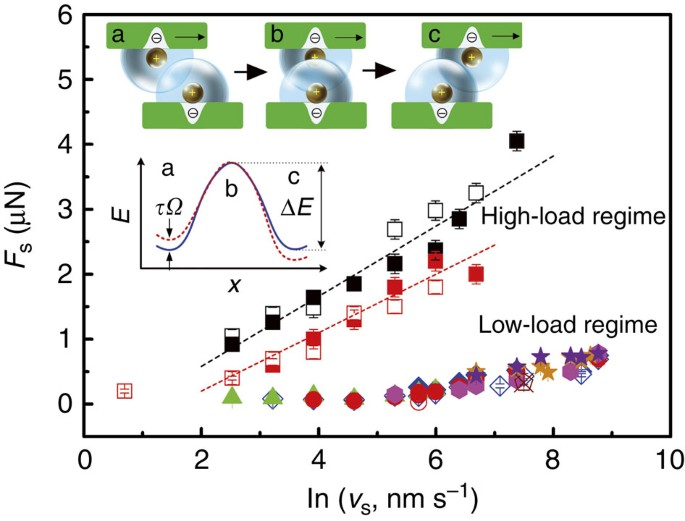Figure 3: Variation of shear forces with sliding velocity.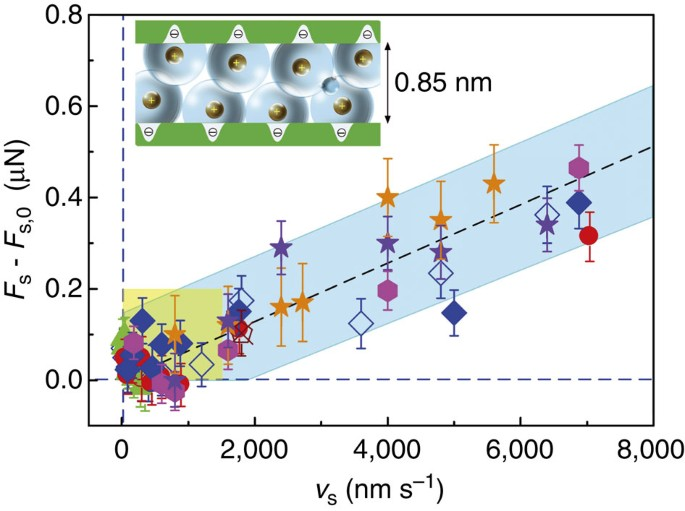Figure 4: Variation of shear forces with sliding velocity in the low-load regime.

## Discussion

Our main findings are that the sliding friction forces vary with sliding velocity either logarithmically or linearly, depending on the surface separation regimes, which in turn depends on the loads (designated high load and low load, respectively). The crucial question is: what are the dominant dissipation modes in these two regimes? The logarithmic variation of the friction with sliding velocity at high loads (Fig. 3) is characteristic of Eyring-like rate-activated processes arising from the overcoming of energy barriers, as seen in a number of solid-friction or boundary lubrication studies21,22. The surface separation Dh=0.48±0.15 nm is comparable with twice the diameter of the bare Na+ ions (~0.2 nm each23,24) localized at the surface charges. Thus, in this regime, the sliding of the two surfaces may be understood in terms of a process where a potential energy ΔE of sliding surface-localized ions past each other needs to be overcome (Methods), as indicated in the scaled cartoon inset in Fig. 3. Energy dissipation occurs predominantly when phonons (degraded into heat) are generated in the irreversible conversion of potential to kinetic energy following the overcoming of the energy maximum25. Such frictional processes may be described by the following relation, derived for this configuration in the Methods section: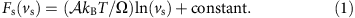Here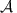is the contact area, kB and T are Boltzmann’s constant and the absolute temperature, and Ω is the stress-activated volume associated with sliding of ions past each other; the constant is linear in ΔE. This mechanism also accounts for the relatively large scatter in Fs in Fig. 2b: the magnitude of Ω and ΔE and thus of the friction force Fs depends on the relative position of the localized counterions on the opposing surfaces sliding past each other. As counterions are localized at the negatively charged lattice sites on the mica surface, the energy barrier to their sliding past each other (Fig. 3 cartoon) depends on the relative orientation of the opposing lattices. Where the relative orientation is sufficiently unfavourable, this may lead to friction large enough to tear the mica surfaces, as happened in several cases (Methods). This relative offset of the opposing lattices was not controlled in our study, and we attribute the scatter in the Fs versus Fn plot in Fig. 2b to such differences between different experiments and contact points. We note that the scatter for a given contact point (where the relative orientation and thus ΔE and Ω are expected to be constant (Methods)) is much lower, as expected (see, for example, the two high-load data sets in Fig. 3).

Although the Fs(νs) behaviour in the high-load regime is dominated by dissipation via activated crossing of energy barriers arising from counterion-localization on the confining surfaces, the behaviour in the lower-load regime, Dl=0.85±0.15 nm, reveals the dissipation in the sheared hydration shells themselves. This is a far more general effect, relevant whenever contacting hydrated species are in relative motion. The variation Fs(νs)–Fs,0=C.νs in this regime, blue band in Fig. 4, comprises a small systematic component Fs,0 arising from indirect coupling of the surfaces to the apparatus (Methods), and a term linearly dependent on the sliding velocity characteristic of viscous dissipation. At the surface separation Dl, the number density ρc/i of counterions trapped between the surfaces is ρc/i=(2σ/Dl), where σ≈2 nm−2 is the areal density of ionizable lattice sites on the mica surface, each of which is associated with a trapped, hydrated Na+ counterion3,18,20,26. This density is determined by the negatively charged sites on the mica, which arise when the K+ ions from the mica surface lattice are lost to solution when it is immersed in water. The number of water molecules nw per Na+ ion trapped in the gap is then given by nw=ρH2O/ρc/i≈6, where ρH2O is the number density of water molecules=(density of water)/(mass of a water molecule). Thus, nw is comparable with the number, 4–7, of water molecules in the first (most tightly held) hydration shell of a Na+ ion23,27. This implies that essentially all the water molecules in the gap Dl≈0.85 nm belong to primary hydration shells of the trapped counterions. This observation enables us to evaluate a dissipation characterized by an effective shear viscosity ηeff of the hydration shells. Applying a Newtonian relation28 to the film, the shear stress may be written as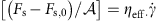, where the shear rate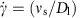. Thus, (∂Fs/∂νs)=(eff/Dl), and from the measured slope C of the blue band in Fig. 4, we may extract the effective viscosity of the confined film, ηeff=0.22±0.07 Pa.s.

This value ηeff of the hydration shell viscosity is some 250-fold larger than the viscosity of bulk water ηH20=8.8 × 10−4 Pa.s at 25 °C. We emphasize that the viscosity of water that is not in hydration layers surrounding a charge, but is likewise confined to subnanometre films by mica surfaces, has an effective value that is comparable to ηH20 (ref. 29) and so is also some two orders of magnitude smaller than ηeff. In other words, it is not the subnanometre confinement that leads to the much higher viscous dissipation of the hydration water, but rather its being within the primary hydration shell surrounding the counterion charges. We also confirmed (see Supplementary Fig. 1 and Supplementary Note 1) that when lower loads are applied so that the equilibrium separation of the surfaces significantly exceeds the value (ca 0.8 nm) at which the confined water molecules all belong to the hydration shells, the effective viscosity within the gap in the linear regime tends—within the scatter of the data—towards that of bulk water.

This measured value of the hydration shell dissipation is consistent with the exchange rate ωH2O,Na+ of water molecules in the hydration shell of Na+ with bulk water molecules23. The value of ωH2O,Na+≈109 s−1 corresponds to an exchange time τH2O,Na+=(1/ωH2O,Na+)≈10−9 s, which may be taken as approximately the relaxation time of water within the hydration shells. This compares with relaxation times τH2O≈10−11 s in bulk water28. On general grounds30, one expects liquid viscosity η to be proportional to molecular relaxation times τ, η= (where G is a limiting high-frequency modulus), as long as the shear rate is much lower than τ−1, as is the case in the present study. The ratio (τH2O,Na+/τH2O)≈100 is thus consistent with the ~250-fold enhancement of the hydration shell viscosity ηeff relative to ηH20. Molecular dynamics simulations of hydrated K+ ions, confined to films of thickness D between mica surfaces31, show an approximately two orders of magnitude enhancement of low-shear-rate viscosity (relative to bulk water) at D=0.94 nm, and are thus also consistent with our findings. Also consistent with our findings is an earlier conjecture made in ref. 3. Likewise, for similarly confined Na+ ions where additional dissipation pathways may be active, the lower limit of values measured in ref. 4, which utilizes a resonance-damping approach, is consistent with our findings for the corresponding surface separation range.

Earlier findings on lubricated sliding between hydrated surface layers including hydrated ions3,4,5, surfactants6,7,8, polymers10,11,12 or liposomes9 could not reveal the underlying process. This is because the measured friction was a convolution of the hydration lubrication with other dissipation pathways, such as the activated processes described above, or deformation6,7,8,9 or entanglement10,11,12 effects in the boundary layers themselves. The present study elucidates this—for the simplest case of trapped, hydrated ions—by unravelling this convolution and separating the dissipation modes (and in future work it would be interesting to examine different aspects such as the case of different ions and of the transition between the regimes). In particular, we identify the dissipation pathway and the viscous losses in the bound hydration layers alone, which are characterized by an effective viscosity orders of magnitude larger than that of non-hydration water. This insight largely accounts for the massive friction reduction via the hydration lubrication mechanism recently described in many systems3,5,6,7,8,9,10,11, and has implications for better design of boundary lubricants and their interfacial vectors in biomedical contexts. Moreover, it provides a framework for understanding lubrication processes in living systems where hydration layers are ubiquitous.

## Methods

### Experimental

Water used was highly purified (Barnstead NanoPure, resistivity 18.2 MΩ cm, total organic content nominally <1 p.p.b.). Salts, used as received, were 99.99% (Merck) and 99.995% (Fluka). The SFB method is described in detail in ref. 32. Contact areas A between the surfaces were evaluated using the Hertzian contact mechanics relation r=(FnR/K)1/3 for the radius of the flattened circular contact of two crossed cylinders, radius R, under a load Fn, where K is an effective mean modulus of the mica/glue layer determined in separate measurements as K=3 × 109 N m−2. The area is then=πr2. Comparison of these values with contact areas obtained by directly measuring the flattening of the optical fringe tips (as in Fig. 2b in the main paper) in the high-load regime were generally within 30% of each other over all experiments. Mean pressures were evaluated as P=(Fn/A). At higher loads (P>ca 1.5 MPa), the mica surfaces often tore on shear, due probably to either trace contamination or, more likely, unfavourable relative orientation of the surfaces (see main text) or a combination of the two, and were aborted following damage. Only experiments where both Fn(D) and Fs profiles were reversible and reproducible (within the scatter) with respect to load and sliding velocities, and thus clearly undamaged, are reported (results are reported from all nine independent experiments in which no damage occurred, that is, different pairs of mica sheets, with different contact points in each; in a substantial number of other experiments, the mica surfaces tore on strong compression and shear, which we attribute to the unfavourable relative orientation of the mica surfaces, see main text and below. In experiments where such damage occurred, no surface interactions could be measured and the experiments were aborted). The weak coupling of the shear springs of the SFB to the body of the apparatus results in a small systematic signal Fs,0 at the drive frequency (considered in ref. 29), and depends on the amplitude Δx0 of lateral motion applied to the top surface (inset to Fig. 1). It is evaluated for each run (of different Δx0) from measurements at large D where the shear force between the surfaces vanishes. The Dh and Dl values are the mean of 23 and 25 measurements in the high-load (ca 5–8 mN) and low-load (ca 0.1–0.2 mN) regimes respectively, where the quoted uncertainty is twice the standard error in the mean given by Peter’s formula33.

### Rate activated sliding

The logarithmic variation of Fs with νs in equation 1 of the main text is similar to analogous relations for sliding but adhering surfaces21,22. Here we sketch a derivation for the configuration inset to Fig. 3, based on a simplified treatment of the Eyring theory of liquid viscosity28.

In the unperturbed system (no shear stress), the frequency ν0 at which a localized ion can overcome the potential barrier ΔE to crossing to an adjacent energy minimum is ν0=νc.exp(−ΔE/kBT), where νc is a characteristic frequency. If a shear stress τ acting on the localized ions is applied to the right, say, the potential is modified by τΩ as indicated by the broken curve in the inset to Fig. 3, where Ω is an effective stress-activated volume. The net rate ν of jumping to the right then becomes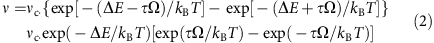where Ω, the effective stress-activated volume, may be much larger than an ionic volume. As long as τΩkBT, we may ignore exp(–τΩ/kBT) relative to exp(τΩ/kBT) in (equation 2), giving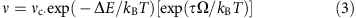The sliding velocity νs is related to the net jump frequency ν, as νs=νd, where d is the mean amplitude of a jump. Substituting for ν=(νs/d) and taking logarithms of both sides, we have finally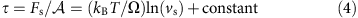giving equation 1 in the text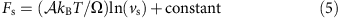(we note that the constant is linear in ΔE)

From the slope ∂Fs/∂(ln(νs))≈0.5 × 10−6 N in Fig. 4, we extract a value for Ω≈2 × 10−23 m3. We can check that indeed τΩ≈3 × 10−20 J>>kBT (≈4 × 10−21 J), which is the assumption underlying in equation 3.